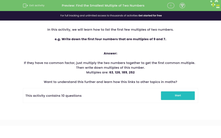# Find the Smallest Multiple of Two Numbers

In this worksheet, students will find the first four lowest common multiples of two given numbers.Key stage:  KS 2

Curriculum topic:   Number: Multiplication and Division

Curriculum subtopic:   Identify Multiples and Factors

Popular topics:   Multiplication worksheets

Difficulty level:#### Worksheet Overview

In this activity, we will learn how to list some common multiples of two numbers.

Example

Write down the first four numbers that are multiples of both 6 and 8.

If we start by listing the multiples of 6 and 8, we can see if there are any common multiples.

Multiples of 6: 6, 12, 18, 24, 30, 36, 42, 48 etc

Multiples of 8: 8, 16, 24, 32, 40, 48 etc.

We can see that we have two common multiples already: 24 and 48.

We don't have to continue listing them all unless we want to because we can use the two that we have found to work out the next ones!

If we find multiples of 24, we know that they will also be common multiples of both 6 and 8!

24 x 2 = 48 which we already know is a common multiple of 6 and 8!

24 x 3 = 72

24 x 4 = 96 etc

So, the first four common multiples of 6 and 8 are 24, 48, 72 and 96.Example 2

Write down the first four numbers that are multiples of both 9 and 7.

If they have no common factor, as in this case, just multiply the two numbers together to get the first common multiple.

9 x 7 = 63

Then write down multiples of this number.

63 x 2 = 126

63 x 3 = 189

63 x 4 = 252

The first four common multiples of 9 and 7 are: 63, 126189252

Let's have a go at some questions now.### What is EdPlace?

We're your National Curriculum aligned online education content provider helping each child succeed in English, maths and science from year 1 to GCSE. With an EdPlace account you’ll be able to track and measure progress, helping each child achieve their best. We build confidence and attainment by personalising each child’s learning at a level that suits them.

Get started